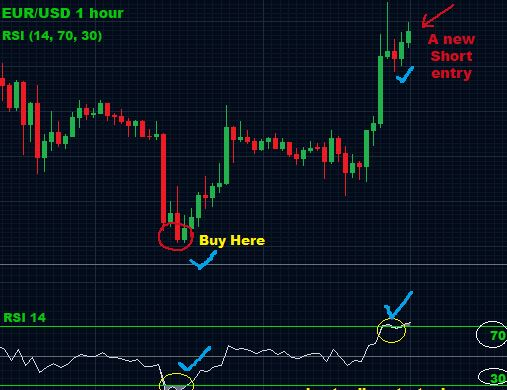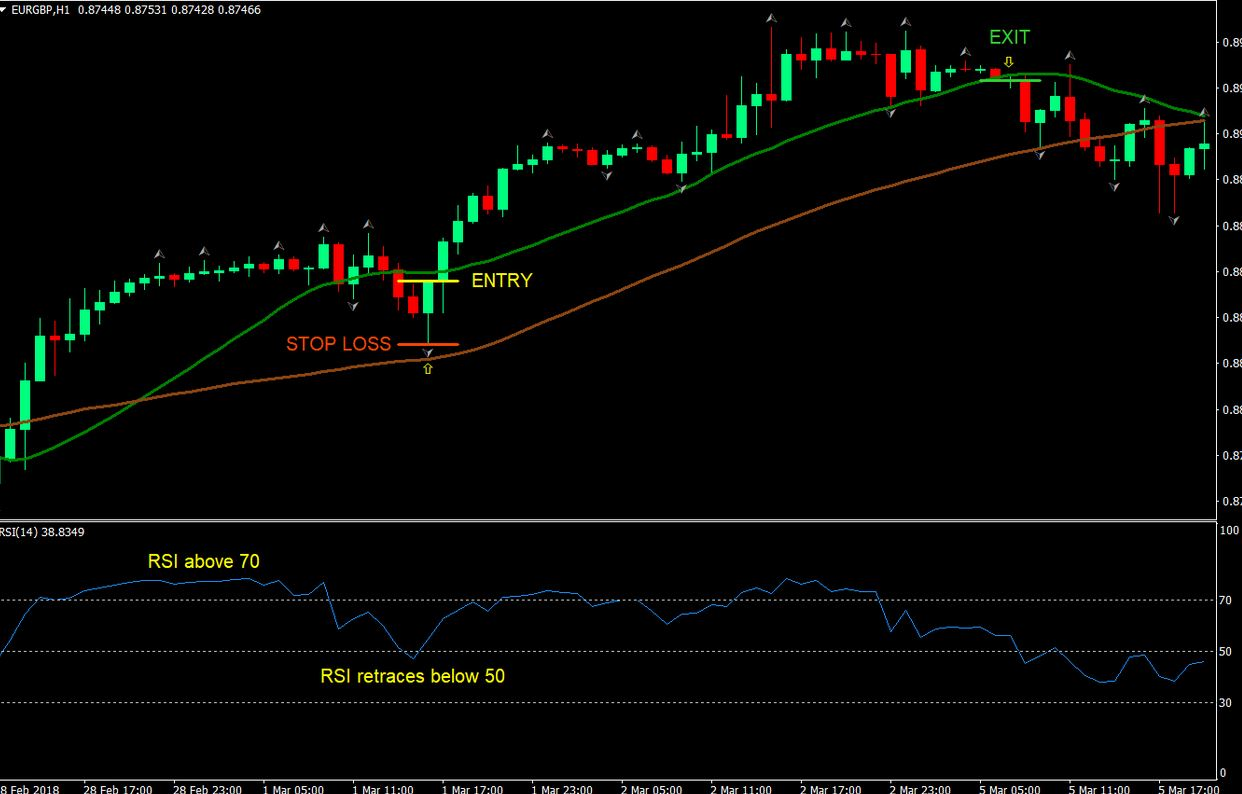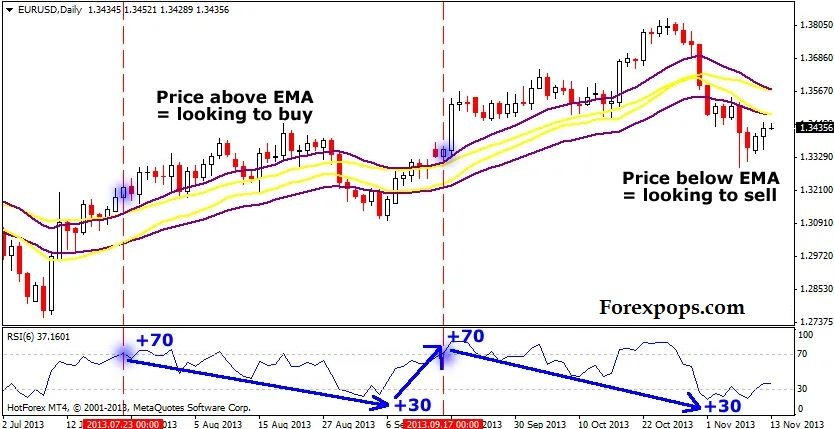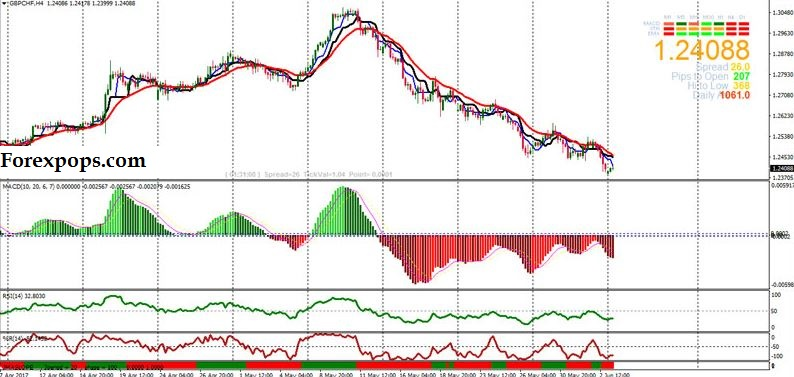The RSI Trading strategies are used to know the performance of a market stock over with the comparison of the earned profits and gained loss. These RSI strategies tells that the what will be the result of the stock if it will be compared with market up and down days. The RSI is a type of oscillator that can display the trading price values with the all previous and current values.

With the help of these values the overbought and oversold values can also be determined. These RSI strategies tell the market RSI trend indicator that either it is up or down also with future estimations. The RSI Trading strategies also tells about the time frames in which the price value ups and downs.

Formula calculation

The results can be generate With this strategy from the given below the formula. The RSI takes the record of the days when the price rate was high and when when the rates were low then this result is matched by the current calculation.Relative Strength = 1.25 (Avg. Gain over last 13 bars) +. 25 (Current Gain) / (.75 (Avg. Loss over last 13 bars) + 0 (Current Loss))

Relative Strength = 1.50 / .75 = 2

RSI = 100 – [100/(1+2)] = 66.67

RSI values calculation

RSI = 100 – (100 / (1 + U/D))

Where:

U – average number of positive price changes

D – average number of negative price changes

Working

The relative strength indicator works best for knowing the overbought and oversold values. In this indicator when the indicator line moves above from 30 to 70 then the stock will for sell and when the indicator line moves below the 30 then the stock will for buy. Always remember it that if the traders will use this method for trading then may be they will get loss. There will be profit from market for the traders.

## MACD Trading Strategy with RSI Indicator MT4

Market trend

The RSI strategies are not only famous for sell and buy signals but this also tells about thebmarket trend and the direction of the trend. This is possible when the RSI ranges are defined. The RSI ranges are  defined into two ways such as bull and bear markets.There are different ranges for both type of markets. For the bull market the range is defined near to the 66.66 that is approximately near to the ratio of 80/20. And for the bear market the range is near to the 33.33 that approximately near to the ratio of 70/30.

RSI Rejection Strategy

Double EMA Channel

Harmonic Pattern Indicator

These are the basically the readings that tells the RSI distance and the signal strength. Mostly traders or witers did not define about these two type of market trends such as bull and bear trend. Mostly they avoid or these are not much common in use but these two trends can be used to define the market trend and trend direction. The RSI strategies are not limited on the only buy signals and sell signals. These strategies can be used for other trading purposes.

## Accurate RSI indicator Buy and Sell Signals MT4/MT5

Up word and Down word Trend

The RSI defines the both trends in both directions like up word direction and the down word direction. As we are already discussed about the RSI ranges above so we will use them to further defining the up word and down word trend.So if the signals will crossed above the 66.66 or 70 then the trend will be in up and also the direction will be upward. But if the the signals will crossed below the 33.33 or 30 then the trend will be in down and also the direction will be down ward.

The RSI ranges are also used for knowing the values of buy and sell and the strength of the buy and sell signals. So how can we know that when the signal will for sell and for buy. There are different scenarios for knowing these signals.

Pivot Point Indicator

Scalping Indicator

If the signals or the indicator line will above from 80 then there are buy signals and the market overbought condition occurs. And  If the signals or the indicator line will down from 20 then there are sell signals and the market oversold condition occurs. The zero point tells the oversold conditions and the 100 point tells the overbought condition. The RSI provides different signals that can be used for buy signals, sell signals, in the form of overbought signal and oversold signals and market up trend signals and market down trend signals.

## Arrow RSI Overbought Oversold Indicator MT4

The RSI breakouts

The RSI strategies are also used for the breakout results. The trend lines can be used for identifying the market breakout results. The RSI strategies tells about the changes in the price. All the dynamic changes can be known through the RSI indicators.

The RSI indicators are well known indicators that are also used for technical analysis. If we use RSI strategies with other trading strategies then the risks and the problems can be betterly handled. The RSI strategies can also be used to know the best future price decisions.There are also some advantages and some key features that defines the elements of the RSI strategies.

1) There are only two level factors in the RSI strategy.

2) This contains only one line.

3) The line ranges are fixed only in between the 0 and 100.

4) The RSI single line can be used for up trend, down trend, up price, down price, overbought and the oversold conditions.